# What is a nonlinear differential equation

## Nonlinear differential equations

### Linearization

The linearization of a differential equation is always a falsification of the mathematical model that represents the differential equation. That is why one can only decide whether the simplification is justified based on the specific problem. Two typical examples from technical mechanics are demonstrated here.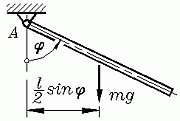Simple pendulum: The pendulum movement of a thin rod is (neglecting movement resistances such as bearing friction and air resistance) by the non-linear initial value problem (chapter "Kinetics of Rigid Bodies")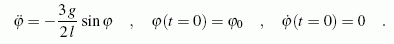(g is the acceleration due to gravity, l the rod length). Points above the variable mean: derivative with respect to time t. The pendulum is at the time t = 0 by the angle φ0 deflected and then released with no initial speed. The free oscillation (without excitation forces or moments) should be considered: The pendulum is deflected and then left to its own devices.

There will be an oscillation with constant amplitude (in each case equal to the initial deflection φ0) to adjust. The time that is required for a full oscillation ("once there and back") depends on the initial deflection. The solution is only possible numerically, you can find it here.

When restricted to "small" initial deflections φ0 the angles remain small and the approximation valid for small angles can be used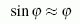to linearize the differential equation. One receives with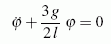a homogeneous linear differential equation of the 2nd order with constant coefficients, which can be solved in closed form. A corresponding differential equation has been solved as an initial example. From the solution, for example, the duration T of a full oscillation can be read off as a formula: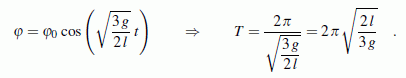It is noteworthy that the oscillation period T is independent of the initial deflection for small pendulum swings. This does not apply to large initial deflections.

Whether the linearization is justified cannot generally be decided (of course it depends on the accuracy requirements). Certainly one can, for example, calculate a pendulum with a maximum deflection of 5 ° with the linearized differential equation.Bending line: The chapter "Deformations due to bending moments" shows that the bending moment is inversely proportional to the radius of curvature of the deformed center of gravity fiber. On this basis, one obtains for the calculation of the deflection v (z) a due to bending moments M.b loaded straight beam the nonlinear differential equation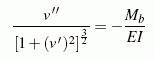(EI is the bending stiffness). This differential equation should actually only be used if extremely large deformations have to be recorded (for example the deformation of the pole of a pole vaulter).

In almost all problems in technical practice, the deflections are very small in relation to the dimensions of the bending beam. Think of the deformations of shafts in mechanical engineering, but also in construction the deformations of bridge girders, for example, are small compared to their dimensions. Therefore it is almost always justified to argue like this:

In the case of very small deformations v (z), the rise in the bending line v 'is also very small, so that the much smaller (v')2 can be neglected compared to 1 (even with an increase of 5 °, 1+ (v ') results in2 = 1+ (tan5 °)2 = 1.00765 an error smaller than 1%). This gives for small deformations with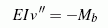the differential equation of the bending line of the 2nd order, which can be used for almost all problems in technical practice with more than sufficient accuracy.